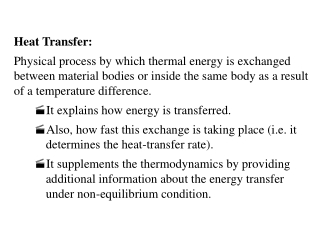DownloadDownload PresentationHeat Transfer:

# Heat Transfer:

Download Presentation## Heat Transfer:

- - - - - - - - - - - - - - - - - - - - - - - - - - - E N D - - - - - - - - - - - - - - - - - - - - - - - - - - -
##### Presentation Transcript

1. Heat Transfer: • Physical process by which thermal energy is exchanged between material bodies or inside the same body as a result of a temperature difference. • It explains how energy is transferred. • Also, how fast this exchange is taking place (i.e. it determines the heat-transfer rate). • It supplements the thermodynamics by providing additional information about the energy transfer under non-equilibrium condition.

2. Conduction Energy transfer from the high-temperature region to the low-temperature region due to thermal diffusion process. It can take place in solids, liquids and gases. The Fourier's law states that the rate at which heat is conducted through a body per unit cross-sectional area is proportional negatively to the temperature gradient existing in the body. where k is the thermal conductivity (W m/ ºC) and dT/dx is the local temperature gradient, dQ/dt is the rate of heat transfer (W), A is the cross-sectional area (m2). Minus sign indicates that the energy flow in the direction of decreasing temperature.

3. Temperature Distribution in a Solid Plate • Questions: • Which junction has higher heat rate? • therefore, heat transfer at section 2 is higher than that at section 1.

4. Temperature Distribution (cont.) • If there is no heat generation or removal, can the temperature inside the block stays constant? If not, what do you think the temperature at the interface will vary? Increase or decrease? • Energy conservation: • Energy accumulation = energy in -energy out • If the energy is flowing out faster than it is flowing in, the total energy inside the block will decrease. Therefore, the energy and, accordingly, the temperature will decrease.

5. Steady Conduction • If temperatures at both surfaces of the block are kept constant, what do you think the temperature distribution inside the block will be after a long period of time? • Given time, it is expected that the temperature distribution inside the block will no longer change with time. Based on the previous argument, the energy in and out should exactly balanced. • Therefore, the temperature profile • should have a constant • slope, that is, it has a linear • distribution.

6. Convection This process of heat transfer from a solid surface to a moving liquid or gas is called convection. The motion of the fluid may be natural (driven by the heated or cooled solid) or forced (driven by external means such as a fan or a pump). The empirical equation governing the convective heat rate is often referred to as the Newton's law of cooling: where the heat is transferred between a surface at uniform temperature Ts and a fluid with reference temperature of T , As is the surface area, and is the mean coefficient of convection heat transfer; the unit for is W/(m2 ° C).

7. Convection (cont.) Convective process is accomplished in two stages: first, heat transfer from solid to fluid through diffusion process adjacent to the surface. Second, the bulk fluid motion will carry transferred heat away from surface. Therefore, the conductivity of the fluid plays an important role in heat convection. It is necessary to study fluid mechanics in order to understand fully the flow aspect of heat convection.

8. Convection (cont. 2)